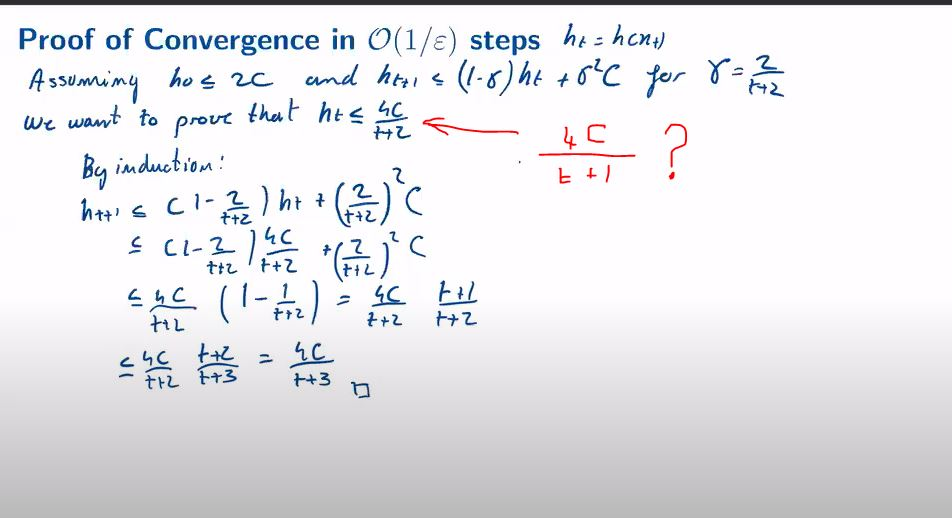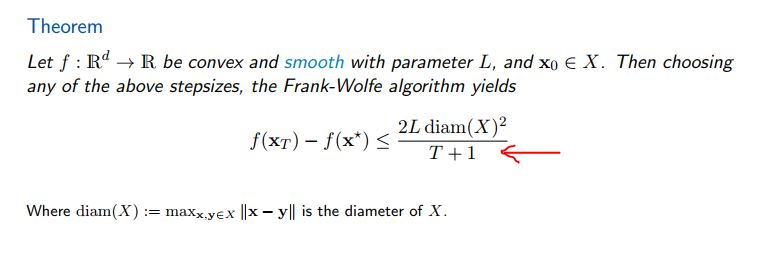### Lecture 8

Hello,
Please correct me if I'm wrong, I think there is a problem on the video of lecture 8 at minute 33, please see the image.
Thanks
AJEGHRIR MustaphaTop comment

Hi Mustapha,

First, the precise choice of the step size will not matter a lot empirically. You can try to implement several of them and you will get the same performance.

The choice of $$\gamma = \frac{2}{t+2}$$ is simply algebraically convenient. The problem with $$\gamma = \frac{2}{t+1}$$ is that it is larger than 1 in the first step (when $$t=0$$ ), and therefore $$1-\gamma \leq 0$$ and the induction will not work.

We could write the upper bound in the theorem as $$\frac{1}{T+2}$$ or $$\frac{1}{T+1}$$ since $$\frac{1}{T+2} < \frac{1}{T+1}$$.

Hi,

The learning rate should be $$\gamma=\frac{2}{t+2}$$. Because $$h_0=2C=\frac{4C}{0+2}$$.

Best,

Yes, but the Theorem wants T+1 not T+2Top comment

Hi Mustapha,

The choice of $$\gamma = \frac{2}{t+2}$$ is simply algebraically convenient. The problem with $$\gamma = \frac{2}{t+1}$$ is that it is larger than 1 in the first step (when $$t=0$$ ), and therefore $$1-\gamma \leq 0$$ and the induction will not work.
We could write the upper bound in the theorem as $$\frac{1}{T+2}$$ or $$\frac{1}{T+1}$$ since $$\frac{1}{T+2} < \frac{1}{T+1}$$.